使用 Unity 实现漂亮的数学曲面(上)

1. 使用Unity的Cube做成一个Prefab
4. C# 逻辑代码，也使用上一篇博客中的完整代码，在本文中会有很多修改

1.2 添加第二个展示函数

$$f(x,t) = (sin(π(x + t)) + \frac{sin(2π(x + t))}{2}) \frac{2}{3}$$

1.3 在编辑中选择函数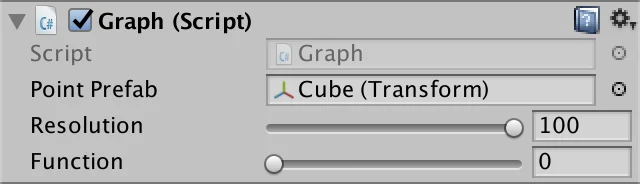1.5 Delegates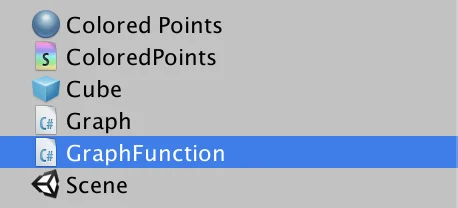1.7 使用枚举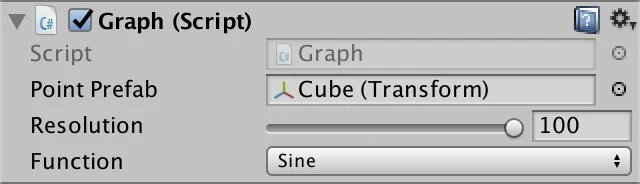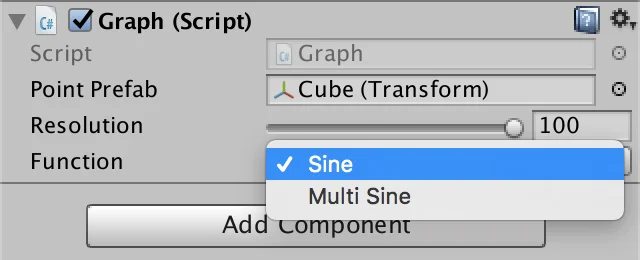2.2 创建网格点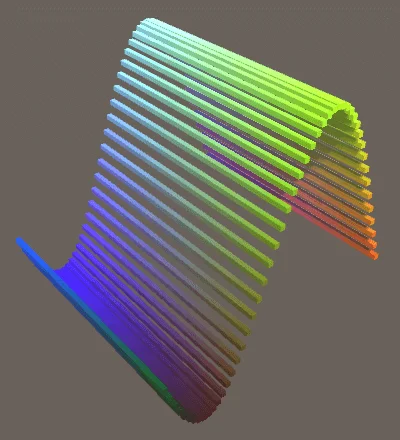2.4 更有趣的效果

$$f(x,z,t) = \frac{sin(π(x + t)) + sin(π(z + t))}{2}$$

Author: iMoegirl